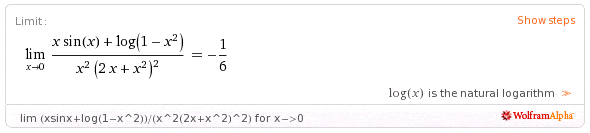# Taylor's series limit 0/0

## Homework Statement

I need to solve this limit in the form 0/0 with the Taylor series...## The Attempt at a Solution

Alright, I didn't really get where I am supposed to "stop" writing polinomials, my teacher said that I should stop when I find the smallest degree factor, because that's the one which is "bossing around" when the limit approaches zero.
Okay, that's where I've gone so far:

http://www4d.wolframalpha.com/Calculate/MSP/MSP619i577b6i0hb357g0000173a0a18c530af8g?MSPStoreType=image/gif&s=64&w=320&h=58 [Broken]

I don't get if I wrote too many, if I didn't write enough terms, if I did something wrong at all, or I am right and should keep on doing calcs. Could someone help me out please? Thanks.

Last edited by a moderator:

D H
Staff Emeritus
Collect like terms in the numerator. What does that give you?

$-(2 x^4)/3-(119 x^6)/120-(1261 x^8)/5040$
OVER
$x^6+4x^5+4x^4$

Even if I collect like terms, I get something that doesn't really get me close to the limit, and I think that huge number there is just wrong...
But, I don't get when I have to stop, I mean, I could have gone for infinity keeping on writing the series polynomials. When do I have to stop writing?

D H
Staff Emeritus
Remember L’ Hôpital’s Rule. If both f(x) and g(x) approach 0 at some point x0, then to evaluate f(x)/g(x) at x0 you try to evaluate f'(x)/g'(x) at x0. If that still results in the indeterminate form 0/0, you can iterate and try to evaluate f''(x)/g''(x). If that doesn't help, try to evaluate f'''(x)/g'''(x), and so on, until you either reach some form that is not indeterminate or a form that blows up.

Assume that you have a Taylor expansion of f(x) and g(x) about the point of interest\begin{aligned} f(x) &= \sum_{n=0}^{\infty} a_n (x-x_0)^n \\ g(x) &=\sum_{n=0}^{\infty} b_n (x-x_0)^n \end{aligned}

where the first few an and bn are zero. (If a0 and b0 are not zero there's no need for this L’ Hôpital rigamarole.)

The limit is
• Zero if the number of leading zeros in {an} is greater than the number of leading zeros in {bn}.
• Undefined (infinite) if the number of leading zeros in {an} is less than the number of leading zeros in {bn}.

These cases are kinda uninteresting. This leaves as an interesting case where the number of leading zeros in {an} and {bn} are equal.

Which case applies to your problem?

Okay, I tried to follow, the result of my limit is neither 0 nor infinity, so it must be when a_n or b_n are equal... uhm... how can I use such information to help myself into the problem?

Dick